# Non-Markovianity and reservoir memory of quantum channels: a quantum information theory perspective

## Abstract

Quantum technologies rely on the ability to coherently transfer information encoded in quantum states along quantum channels. Decoherence induced by the environment sets limits on the efficiency of any quantum-enhanced protocol. Generally, the longer a quantum channel is the worse its capacity is. We show that for non-Markovian quantum channels this is not always true: surprisingly the capacity of a longer channel can be greater than of a shorter one. We introduce a general theoretical framework linking non-Markovianity to the capacities of quantum channels and demonstrate how harnessing non-Markovianity may improve the efficiency of quantum information processing and communication.

## Introduction

The description of quantum systems interacting with their environment is the central objective of the theory of open quantum systems1. During the last few years there has been an increasing interest in open quantum systems with memory, also known as non-Markovian open quantum systems2,3,4,5,6,7,8,9,10,11,12, due to both fundamental and applicative reasons. From a fundamental point of view the study of quantum systems interacting with structured environments, while presenting considerable difficulties from a theoretical point of view, is of crucial importance for the realistic description of a variety of physical systems such as photonic band gap materials, quantum biological systems and complex quantum networks, solid state systems (e.g., SQUIDs and Josephson junctions) and ultracold gases. From an applicative point of view, the increasing ability in reservoir engineering techniques paves the way to new methods of decoherence control based on the manipulation and modification of properties of the environment such as its frequency spectrum13,14,15.

Non-Markovianity is a multifaceted and complex phenomenon that cannot be simply grasped by looking at specific instances and cannot be generally traced back to a single unique feature of the environment. Several measures of non-Markovianity have been introduced, based on distinguishability of quantum states as measured by trace distance3 or fidelity4, semigroup property5 or divisibility6 of the dynamical map, Fisher information7, or quantum mutual information8. In general these measures or witnesses do not coincide and examples of differences between them have been reported even for simple open quantum systems16. This is an obvious consequence of the fact that reservoir memory may have different effects on different dynamical properties that one may want to harness for certain specific purposes. Rather than being a problematic aspect, we believe that this richness and variety constitutes the power of non-Markovian open quantum systems.

Very recently the role of structured environments and non-Markovianity in quantum metrology17, quantum key distribution18, quantum teleportation19, entanglement generation20, optimal control21 and quantum biology22,23, has started to be investigated, showing with increasing evidence that non-Markovian quantum channels may be advantageous compared to Markovian ones. However, to date there is no general theory linking non-Markovian dynamics with an increase in the efficiency of quantum information processing and communication24. This is exactly the scope of this Letter. Our main result is the identification of specific features of non-Markovianity that lead to an increase in the capacities of quantum channels compared to the corresponding Markovian ones. We emphasize the overlooked connection between the data processing inequality and the divisibility of the dynamical map and explore this relation to reveal the key signature of non-Markovianity. Our approach establishes a link between the reservoir memory effects and the variation in the entropy of the system and of the environment, paving the way to a description of reservoir memory in terms of information flow and exchange between the system and the environment.

## Results

### Quantum capacities

The general scenario typical of quantum information processing and communication sees Alice and Bob at the opposite ends of a quantum channel, the former sending information (classical or quantum) and the latter receiving it. The maximum amount of information that can be reliably transmitted along a noisy quantum channel is known as the channel capacity. In this Letter we will be concerned with two types of capacities, the entanglement-assisted classical capacity Cea and the quantum capacity Q25. The first quantity sets a bound on the amount of classical information that can be transmitted along a quantum channel when one allows Alice and Bob to share an unlimited amount of entanglement. It is defined in terms of the quantum mutual information I(ρ, Φt) between the input and the output of the channel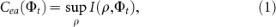where I(ρ, Φt) = S(ρ) + Stρ) − S(ρ, Φt), with S(ρ) = −tr(ρ ln ρ) the von Neumann entropy of the input state, Stρ) the entropy of the output state andthe entropy exchange, i.e., the entropy at the output of the complementary channel25. We indicate with Φt the dynamical map describing the quantum evolution of a generic open quantum system. The dynamical map defines a family of quantum channels for t ≥ 0, such that. If ρ is the initial state of the system, then Φtρ defines the state at time t that tells us for how long the quantum state, encoding classical or quantum information, is subjected to environmental noise. In experimental implementations of quantum protocols, e.g. with trapped ion systems, t is the duration of the experiment and it is obviously connected to the length of the channel, i.e. the length of an optical fiber in optical systems. The quantum capacity Q, on the other hand, gives the limit to the rate at which quantum information can be reliably sent down a quantum channel. For single use of the channel, it is defined in terms of the coherent information Ic(ρ, Φt) as follows27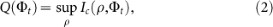with Ic(ρ, Φt) = Stρ) − S(ρ, Φt). More in general, the quantum channel capacity is defined as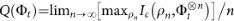, where ρn is the density matrix describing the sequence of states sent through the channel and the maximum is performed over all input states of n successive channel uses25. We note that, contrarily to the entanglement-assisted classical capacity, the quantum channel capacity is in general not additive. However, for degradable channels28, the general definition coincides with Eq. (2) and additivity holds.

### Capacity-based non-Markovianity measures

One of the central results of quantum information theory is the quantum data processing inequality29 which, intuitively, says that processing quantum information reduces the amount of correlations between input and output. More precisely, given the quantum channels,and their concatenation, we have. For divisible channels Φt = Φt,sΦs, with st, the data processing inequality implies Ic(ρ, Φt) ≤ Ic(ρ, Φs). A similar inequality holds for the mutual information, i.e. I(ρ, Φt) ≤ I(ρ, Φs). As a consequence, for divisible quantum channels, both the entanglement-assisted classical capacity Ceat) and the quantum capacity Qt) decrease monotonically with time. There exist, however, physical dynamical maps that cannot be written as concatenation of two completely positive trace preserving (CPTP) channels Φt,s and Φs. For these systems, the coherent information and the mutual information may not simply decrease monotonically in time. Here we claim that this very concept is at the basis of the information theoretic approach to non-Markovianity.

It is worth stressing that throughout the paper we discuss non-Markovian dynamics as arising from the interaction between the system and a physical environment with certain spectral features. However, we assume that the channel Φt is memoryless in the sense of the definition typically used in quantum information theory26. More precisely, one should remember that when describing information transmission one considers not just the dynamics of a single system, but of sequences of systems each interacting with the environment. Here we assume that the environment acts identically and independently on each element of the sequence, so in this sense the channel is memoryless. However, we assume that the dynamics of each system in the sequence is non-Markovian due to non-negligible system-environment correlations. In this typical open quantum systems scenario we associate the dynamical non-Markovianity to reservoir memory effects1.

All existing measures of non-Markovianity3,4,5,6,7,8 are based on non-monotonic behaviour of certain quantities occurring when the divisibility property is violated. Following the same line of reasoning, we define here two new measures of non-Markovianity based on the non-monotonic behavior of the quantum and entanglement-assisted classical capacities,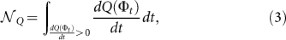and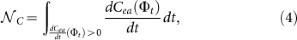where the integrals above are extended to all time intervals over which dQ/dt and dCea/dt are positive.

Our new measures allow for the interpretation of reservoir memory effects in terms of back flow of the maximum amount of quantum (classical) accessible information on the initial state, after the state has been subjected to a noisy channel for a certain time. Remarkably, since both coherent information and quantum mutual information connect the entropy of the open quantum system Stρ) (hence its information content) to the variation in entropy of the environment S(ρ, Φt), our measures effectively describe reservoir memory in terms of exchange of information, or information flow, between system and environment. This is not the case for the other non-Markovianity measures3,4,5,6,7 where a link between information on the system and on the environment does not exist.

It it worth noting that the two measures of non-Markovianity introduced above, in general, do not coincide even for degradable channels and in fact they distinguish between two different types of resources, being related to revivals of correlations which can be used to transfer either classical information or quantum information down a quantum channel.

Generally, non-Markovianity measures quantify a property of the dynamical map and are, therefore, defined by optimizing over all initial states of the system. This optimization problem makes it virtually impossible to evaluate (even numerically) their value for more than 2 qubits. As the effects of decoherence notably increase dramatically for increasing qubits numbers n, it is crucial to be able to evaluate the advantages of non-Markovianity for any n. A very important feature ofandis that, due to the additivity of Q (for degradable channels) and Cea, they satisfy the property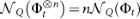, which allows us to calculate straightforwardly the non-Markovianity measure of n qubits in identical uncorrelated environments from the non-Markovianity measure of a single qubit.

An analysis of non-Markovianity in the framework of resource theory is beyond the scope of this Letter. However, we claim here that non-Markovianity can be a resource in the sense that it may allow tasks (reliably transmitting information for longer distances) that are impossible in its absence. We take here a more physical approach than the general information theoretic one. We indeed start from the assumption that every quantum system interacts with a physical environment, whose characteristics depend on the specific physical context considered. The presence of the environment is unavoidable, but it is possible to modify some of its properties, e.g. its frequency spectrum, by means of reservoir engineering techniques. In this context Markovian dynamics, i.e., exponential decay of population or coherences, is always an approximation of the exact dynamics. Such an approximation, nonetheless, describes accurately the time evolution on a coarse grained time-scale, for certain open quantum systems. By means of reservoir engineering we can induce non-Markovianity in time scales or distances appreciable in the experiments. More precisely, in this way one can change an otherwise Markovian dynamics into a non-Markovian one and, as a consequence, find better conditions for quantum communication purposes as measured by channel capacities.

Markovian dynamical maps, characterized by constant positive decoherence rates, generally lead to irretrievable deterioration of the channel capacity as the length of the channel increases. On the contrary non-Markovian dynamical maps charactertized by time-dependent decoherence rates may lead to (i) increase of the channel capacities for a given channel length, (ii) revivals of the channel capacities, hence increasing the values of channel lengths over which the capacities are non zero, (iii) length-independent finite-capacity channels (residual channel capacity), i.e., channels for which the quantum and/or entanglement-assisted classical capacity remains unchanged and positive, after a certain threshold length.

In order to compare Markovian and non-Markovian channel capacities a few important remarks are in order. In the spirit of reservoir engineering, when we talk of Markovian dynamical map corresponding to a non-Markovian one, we mean the map obtained for values of the system and environment characteristic parameters such that reservoir memory effects are negligible after a short initial time scale. This is the regime in which a coarse grained time-evolution would be correctly describe by a Markovian dynamical semigroup. Often this Markovian regime requires a weak system-reservoir coupling assumption. Hence, the coherences are initially maintained for longer compared to the non-Markovian regime in which the coupling is stronger. However, they always inevitably decay exponentially while in the non-Markovian case, due to reservoir memory effect, phenomena (i)–(iii) may occur. Hence there will often be a threshold after which the channel capacities in the non-Markovian regime are higher than in the corresponding Markovian regime.

It is worth noticing, however, that dynamical maps arising from a microscopic description do not necessarily admit a Markovian limit for all values of the parameters. This, e.g., occurs in some of the examples considered below. Hence, generally, our focus will be on showing how reservoir engineering allows to improve quantum communication protocols, pointing out if and when this is connected to information back flow as indicated byor.

In the following we will illustrate these points by looking at three exemplary types of exact and therefore non-Markovian, quantum channels: the dephasing channel, the amplitude damping channel in a Lorentzian environment and the amplitude damping channel in a photonic band gap.

### Example 1 - pure dephasing

Let us begin by considering the dephasing channel for a qubit described by a master equation with dissipator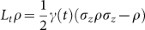, with γ(t) the time-dependent decoherence rate. The density matrix evolves in time according to the equations ρij(t) = ρije−Γ(t) and ρii(t) = ρii,

whereand ρij are the density matrix elements of the initial state ρ.

Note that γ(t) needs not be positive. If γ(t) ≥ 0, then the channel is divisible.

The dephasing channel is degradable for all admissible γ(t), i.e. whenever Γ(t) ≥ 0. This simplifies the calculations of the quantum capacity. Indeed, we find that Q takes a simple analytical formulae28, i.e., QD(t) = 1 − H2[(1 + e−Γ(t))/2], with H2[.] the binary Shannon entropy. Since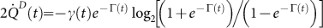the measureis non-vanishing if and only if γ(t) < 0, i.e., whenever the dynamical map is non-divisible. A similar calculation for the entanglement-assisted classical capacity shows that. It follows immediately that. For dephasing channels all known measures of non-Markovianity vanish if and only if the channel is divisible.

In Fig. 1 we show the behavior of the quantum channel capacity QD as a function of time or, equivalently, of the channel length for the exact model of dephasing of Ref. 30, with Ohmic reservoir spectrum of the form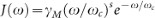, with ωc the cutoff frequency, γM the coupling constant and s the Ohmicity parameter. For zero temperature bosonic environments the dynamical mapis divisible if and only if 0 < s ≤ 231. We can change the non-Markovian character of the channel by changing the Ohmicity paramter s. This can be experimentally implemented, e.g., with impurities in ultracold atomic gases as demonstrated in Ref. 32.

Note that in this system the dynamics does not admit a Markovian limit for s ≥ 1 as, in this case, the dephasing rate γ(t) vanishes for. On the contrary, for 0 < s < 1, after a time of the order of, the dephasing rate reaches a constant positive value and the coherences start decaying exponentially. In Fig. 1 we compare the non-Markovian dynamics obtained for s = 3 (blue line) to the case s = 1 where the system behaves in a Markovian way for ωct > 1 (red line). We see here that, for longer times the non-Markovian case is preferable over the Markovian one and that the latter leads to vanishing Qd. We highlight two important features of the non-Markovian character of the quantum channel, namely, the non-monotonicity of QD, which initially decreases with time but then starts increasing again after a certain threshold value of time or channel length and the existence of residual quantum channel capacity. The connection between stationary coherences leading to channel capacity trapping and non-Markovianity has been discussed in Ref. 33.

The dephasing model here discussed can be implemented experimentally in the context of ultracold atomic gases. In Ref.34 the pure dephasing master equation is derived starting from a microscopic system-environment model describing cold atoms in superlattices (open system) immersed in a harmonically trapped Bose-Einstein condensate (BEC) acting as an environment. Collisions between the BEC atoms and the atoms in the optical lattice lead to decoherence phenomena that, under certain conditions, can be described as pure dephasing. More precisely purely dephasing dynamics occurs when the BEC can be considered homogeneous (shallow harmonic trap) and weakly interacting and when tunnelling between the two wells forming the double-well potential of the super-lattice is neglected. Non-Markovianity in this model has been studied in Ref. 32 where it has been shown that by changing the BEC scattering length and the dimensionality of the gas one can engineer different types of both Markovian and non-Markovian environments. Generally increasing the BEC scattering length, i.e., the strength of interactions within the BEC, leads to an increase in non-Markovianity of the system. The low frequency part of the spectrum in this system is of the Ohmic form with s = 1 + D for weakly interacting gases, with D the dimensionality of the BEC34. Since here s ≥ 2 always, in this system we will always observe the onset of stationary coherences leading to the channel capacity trapping observed in Fig. 1.

### Example 2 - amplitude damping

The second example we consider is the qubit amplitude damping channeldescribed by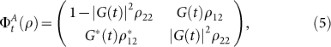where G(t) satisfies the non-local equation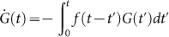with initial condition G(0) = 1 and f(t) is the reservoir correlation function which is related via Fourier transform to the spectral density J(ω). This model describes the dissipative interaction between a two-level system and a zero-temperature bosonic reservoir, leading to an exact master equation of the form1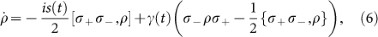withand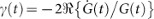the time-dependent Lamb shift and decay rate, respectively and σ± the spin inversion operators.

The amplitude damping channel is degradable for, while foris anti-degradable with zero quantum capacity. The states optimizing Ic(ρ, Φt) and I(ρ, Φt) are now time-dependent, but the optimization problem is still solvable. One finds35,36 the following formulae for the entanglement assisted capacity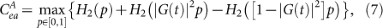and the quantum capacity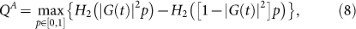for(otherwise).

#### Lorentzian Spectrum

We begin by considering a Lorenztian spectrum of the form J(ω) = γMλ2/2π[(ω − ωc)2 + λ2], with λ the width of the Lorentzian, ωc its peak frequency and γM an effective coupling constant. In the resonant case δ = ω0 − ωc = 0, with ω0 the qubit frequency, the function G(t) takes a simple form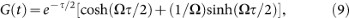with, R = γM/λ and τ = λt. In the weak coupling regime, i.e. for 2R ≤ 1, G(t) is monotonically decreasing, whereas in the strong coupling regime, 2R > 1, G(t) is oscillating so there are periods whenis positive. It is straightforward to show that γ(t) ≥ 0 for 2R ≤ 1, while it can take temporarily negative values for 2R > 1. In the latter case the dynamical map is not divisible. However, due to the fact that the amplitude damping channel is anti-degradable for 2|G(t)|2 < 1, from a quantum information processing point of view, only revivals that occur in the region 2|G(t)|2 > 1 are important.

This example is very useful to illustrate the subtleties of the comparison between the Markovian and the non-Markovian regimes. To reduce the non-Markovian character of the map we can use two strategies (or more generally a combination of them). The first and obvious one is to consider the limit, corresponding to a flat and weakly coupled spectrum. If we compare this Markovian regime, for, to the non-Markovian one obtained when, we will find that generally the channel capacities are higher in the Markovian case. This is, however, obvious. The more we reduce the coupling with the environment, the better is the resulting channel for quantum communication purposes. A more interesting situation occurs if we assume that we cannot change λ/γM but we can instead change the detuning δ. In this case, the more detuned is the system, the longer the qubit populations tend to oscillate. As a consequence non-Markovianity can increase for increasing detunings, leading to better and better values of the quantum channel capacity, as shown in Fig. 2. More precisely the increased values of Q(t) for increased detunings are due to both a reduction in the amount of information lost into the environment (smaller effective coupling to the environment) and to an increase in the information backflow as indicated by the appearance of new oscillations. In this sense non-Markovianity, describing information backflow, certainly plays a role in the behaviour of the quantum channel capacity displayed in Fig. 2.

It is interesting to highlight the differences between the entanglement-assisted capacityand the quantum capacity QA in the amplitude damping model here considered. In Fig. 3 we plotand QA for two different values of R in the strong (R = 10) and ultra-strong (R = 100) coupling regimes. For these values of parameters the dynamical map is always non-divisible and, hence, all the previously introduced non-Markovianity measures3,5,6,7,8,9 are non-zero. However, in Fig. 3 we see that, while Cea always exhibits revivals, i.e.decreases monotonically eventually vanishing for R = 10, where. Revivals ofoccurs only in the ultra-strong coupling regime. This example shows the difference between the two capacity-based measures introduced in this Letter. The careful reader will not be surprised by this result. We should expect indeed that the transmission of quantum information along a quantum channel is more sensitive to noise than the transmission of classical information (although assisted by entanglement shared between Alice and Bob).

#### Photonic Band Gap

Also the second example of amplitude damping channel stems, as the previous one, from an exact microscopic model of an open quantum system. The environment is a bosonic zero temperature three-dimensional periodic dielectric with isotropic photon dispersion relation. In this ideal photonic crystals, a photonic band gap is the frequency range over which the local density of electromagnetic states and the decay rate of the atomic population of the excited state vanish. Near the band gap edges the density of states becomes singular, the atom-field interaction becomes strong and one can expect modifications to the spontaneous emission decay. We consider the model described in Ref. 37. In this case the function |G(t)|2 depends on two relevant parameters, the detuning δ = ω0 − ωe from the band gap edge frequency ωe and the parameter β defined as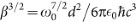withthe Coulomb constant and d the atomic dipole moment. Population trapping is a general feature of this model. The farther the atomic transition frequency is from ωe and inside the gap, the higher is the fraction of initial state population which is trapped in the excited state for t → ∞. This in turn gives rise to stationary values of the quantum and classical capacities as we can see for QA in Fig. 4. The opposite regime is when the qubit frequency falls outside the gap and far from the edge ωe, in which case a fast Markovian exponential decay typical of atomic spontaneous emission occurs and the channel capacities vanish very rapidly. Here it is clear that, for this system, increasing non-Markovianity one obtains better an better values of channel capacities because of the increasingly effective population trapping, as shown in Fig. 4.

We conclude noticing that for amplitude damping channels the non-Markovianity measures associated to Q and Cea, defined in Eqs. (3)–(4), are not simply related. In generaland the entanglement-assisted classical capacity shows similar features to the ones that we have seen for the quantum capacity. Finally, we note that, for the amplitude damping channel, there exist values of parameters for which the non-Markovianity measures of Refs. 3,6 are non zero, but still, proving that these measures in general are not equivalent.

## Discussion

In the quest for realistic large scale implementations of quantum devices for quantum technologies one of the major existing challenges is the identification of ways to increase both the distance over which quantum information is reliably transferred and distributed and the coherence times of quantum information processing. The results presented in this article demonstrate that careful manipulation of the environmental properties based on the exploitation of environmental memory effects and non-Markovianity can be generally used to induce revivals of classical and quantum capacities as well as for engineering distance (or time)-independent values of these quantities. This means that, in certain situations, reservoir memory effects may be exploited to improve realistic error correction schemes working for any channel length. In this sense our results prove that non-Markovianity is a new and yet unexplored resource for quantum technologies, with the potential to pave the way to real-scale quantum-enhanced devices.

## Methods

### Dephasing Channel: Dynamical map and its capacity

The dephasing channel (dynamical map)for a qubit is described by the local in time master equation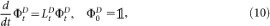with the following local generator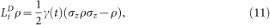and time-dependent dephasing rate γ(t).

This generator and the corresponding master equation, can be derived exactly from the following microscopic Hamiltonian description of system (noisy channel) plus environment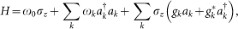with ω0 the qubit frequency, ωk the frequencies of the reservoir modes,the annihilation (creation) operators of the bosonic environment and gk the coupling constant between each reservoir mode and the qubit. In the continuum limit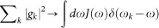, where J(ω) is the reservoir spectral density.

The action of the dynamical mapon a qubit is described by the following formula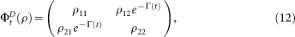where ρij are the density matrix elements of the initial state ρ. One easily finds the operator-sum representation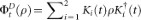with the time-dependent Kraus operators:and, where. While γ(t) may temporarily attain negative values, complete positivity of the dynamical map imposes that Γ(t) ≥ 0. We note in passing that the usual Markovian quantum channel for pure dephasing can be written in terms of Kraus operators having the same operatorial form as those reported above, provided one replaces Γ(t) with γMt.

Using the Kraus operators one can write the complementary map, needed to calculate both the coherent information and the entropy exchange which appears in the definition of the mutual information of the channel, as follows: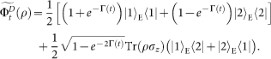The dephasing channel is degradable for all values of Γ(t), which simplifies the calculations of the quantum capacity. In this case, indeed, we find that the state optimizing the coherent information in the definition of the quantum capacity does not depend either on time or on the specific properties of the environmental spectrum. Having this in mind one can show that Q takes the following simple analytical formula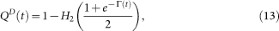with H2(.) the binary Shannon entropy. Since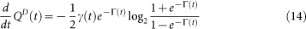the measurehas nonzero value if and only if γ(t) < 0, i.e., whenever the dynamical map Φt is not divisible.

### Amplitude damping channel: Dynamical map and its capacity

The amplitude damping channelis described by the exact local generator given in Eq. (6). The local generator and corresponding master equation can be derived exactly by the following microscopic Hamiltonian model describing a two-state system interacting with a bosonic quantum reservoir at zero temperature1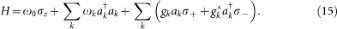The generator of the Markovian amplitude damping channel has the same form of Eq.(6), provided that one replaces the time dependent coefficients s(t) and γ(t) with positive constant values sM and γM. It is worth noticing that Markovian quantum channels are always approximations of exact (and therefore non-Markovian) ones, hence they generally describe only a restricted number of physical systems and processes for which these approximations are valid.

The Kraus representation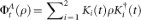for the amplitude damping channel is given byand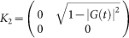which gives us a complementary map defined by: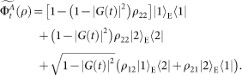From the expression of the complementary map one obtains the entanglement assisted and quantum channel capacities of Eqs. (7)–(8).

If the reservoir spectral density has a Lorenztian shape, i.e. J(ω) = γMλ2/2π[(ω − ωc)2 + λ2], then the function G(t) takes the form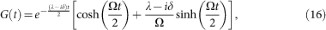withwhere w = γMλ/2 + δ2/4 and δ = ω0 − ωc. For δ = 0, one obtains Eq. (16).

## References

1. Breuer, H.-P. & Petruccione, F. The Theory of Open Quantum Systems (Oxford Univ. Press, 2007).

2. Piilo, J., Maniscalco, S. & Suominen, K.-A. Non-Markovian quantum jumps. Phys. Rev. Lett 100, 180402 (2008).

3. Breuer, H.-P., Laine, E.-M. & Piilo, J. Measure for the degree of non-Markovian behavior of quantum processes in open systems. Phys. Rev. Lett. 103, 210401 (2009).

4. Vasile, R., Maniscalco, S., Paris, M. G. A., Breuer, H.-P. & Piilo, J. Quantifying non-Markovianity of continuous-variable Gaussian dynamical maps. Phys. Rev. A 84, 052118 (2011).

5. Wolf, M. M. et al. Assessing non-Markovian quantum dynamics. Phys. Rev. Lett. 101, 150402 (2008).

6. Rivas, A., Huelga, S. F. & Plenio, M. B. Entanglement and non-Markovianity of quantum evolutions. Phys. Rev. Lett. 105, 050403 (2010).

7. Lu, X.-M., Wang, X. & Sun, C. P. Quantum Fisher information flow and non-Markovian processes of open systems. Phys. Rev. A 82, 042103 (2010).

8. Luo, S., Fu, S. & Song, H. Quantifying non-Markovianity via correlations. Phys. Rev. A 86, 044101 (2012).

9. Lorenzo, S., Plastina, F. & Paternostro, M. Geometrical characterization of non-Markovianity. Phys. Rev. A 88, 020102 (2013).

10. Chruściński, D. & Kossakowski, A. Non-Markovian Quantum Dynamics: Local versus Nonlocal. Phys. Rev. Lett. 104, 070406 (2010).

11. Liu, B.-H. et al. Experimental control of the transition from Markovian to non-Markovian dynamics of open quantum systems. Nature Phys. 7, 931–934 (2012).

12. Chruściński, D. & Maniscalco, S. Degree of Non-Markovianity of Quantum Evolution. Phys. Rev. Lett 112, 120404 (2014).

13. Biercuk, M. J. et al. Optimized dynamical decoupling in a model quantum memory. Nature 458, 996 (2009).

14. Myatt, C. J. et al. Decoherence of quantum superpositions through coupling to engineered reservoirs. Nature 403, 269273 (2000).

15. Verstraete, F., Wolf, M. M. & Cirac, J. I. Quantum computation and quantum-state engineering driven by dissipation. Nature Phys. 5, 633636 (2009).

16. Haikka, P., Cresser, J. D. & Maniscalco, S. Comparing different non-Markovianity measures in a driven qubit system. Phys. Rev. A 83, 012112 (2011).

17. Chin, A. W., Huelga, S. F. & Plenio, M. B. Quantum Metrology in Non-Markovian Environments. Phys. Rev. Lett. 109, 233601 (2012).

18. Vasile, R., Olivares, S., Paris, M. G. A. & Maniscalco, S. Continuous variable quantum key distribution in non-Markovian channels. Phys. Rev. A 83, 042321 (2011).

19. Laine, E., Breuer, H.-P. & Piilo, J. Nonlocal memory effects allow perfect teleportation with mixed states. arXiv 1210.8266 (2012).

20. Huelga, S. F., Rivas, A. & Plenio, M. B. Non-Markovianity-Assisted Steady State Entanglement. Phys. Rev. Lett. 108, 160402 (2012).

21. Schmidt, R., Negretti, A., Ankerhold, J., Calarco, T. & Stockburger, J. T. Optimal Control of Open Quantum Systems: Cooperative Effects of Driving and Dissipation. Phys. Rev. Lett. 107, 130404 (2011).

22. Thorwart, M. et al. Enhanced quantum entanglement in the non-Markovian dynamics of biomolecular excitons. Chem. Phys. Lett. 478, 234–237 (2009).

23. Chin, A. W., Prior, J., Rosenbach, R., Caycedo-Soler, F., Huelga, S. F. & Plenio, M. B. The role of non-equilibrium vibrational structures in electronic coherence and recoherence in pigmentprotein complexes. Nature Phys. 9, 113–118 (2013).

24. Nielsen, M. A. & Chuang, I. L. Quantum Computation and Quantum Information (Cambridge University Press, 2000).

25. Holevo, A. S. & Giovannetti, V. Quantum channels and their entropic characteristics. Rep. Prog. Phys. 75, 046001 (2012).

26. Caruso, F., Giovannetti, V., Lupo, C. & Mancini, S. Quantum channels and memory effects. arXiv 1207.5435 (2012).

27. Loyd, S. The Capacity of a Quantum Channel for Simultaneous Transmission of Classical and Quantum Information. Phys. Rev. A 55, 16131622 (1997).

28. Devetak, I. & Shor, P. W. The Capacity of a Quantum Channel for Simultaneous Transmission of Classical and Quantum Information. Commun. Math. Phys. 256, 287–303 (2005).

29. Schumacher, B. & Nielsen, M. A. Quantum data processing and error correction. Phys. Rev. A 54, 26292635 (1996).

30. Palma, G. M., Suominen, K. A. & Ekert, A. K. Quantum computers and dissipation. Proc. R. Soc. Lond. A 452, 567 (1996).

31. Haikka, P., Johnson, T. & Maniscalco, S. Time Invariant Discord and Non-Markovianity. Phys. Rev. A 87, 010103(R) (2013).

32. Haikka, P. et al. Quantifying, characterizing and controlling information flow in ultracold atomic gases. Phys. Rev. A 84, 031602(R) (2011).

33. Addis, C., Brebner, G., Haikka, P. & Maniscalco, S. Coherence trapping and information backflow in dephasing qubits. Phys. Rev. A 89, 024101 (2014).

34. Cirone, M. A., De Chiara, G., Palma, G. M. & Recati, A. Collective decoherence of trapped atoms coupled to a Bose-Einstein condensate. New J. Phys. 11, 103055 (2009).

35. Giovannetti, V. & Fazio, R. Information-capacity description of spin-chain correlations. Phys. Rev. A 71, 032314 (2005).

36. Benenti, G., D' Arrigo, A. & Falci, G. Enhancement of Transmission Rates in Quantum Memory Channels with Damping. Phys. Rev. Lett. 103, 020502 (2009).

37. John, S. & Quang, T. Spontaneous emission near the edge of a photonic band gap. Phys. Rev. A 50, 17641769 (1994).

## Acknowledgements

The authors thank Shashank Virmani, Chiara Macchiavello and Pinja Haikka for valuable discussions on the topic of the Letter. B.B. thanks the Institute of Photonics and Quantum Sciences at Heriot-Watt University, Edinburgh, where part of this work was done, for the hospitality and acknowledges financial support form the Finnish Emil Aaltonen Foundation and the National Science Center project 2011/01/N/ST2/00393. D.C. was partially supported by the National Science Center project DEC-2011/03/B/ST2/00136.

## Author information

B.B, D.C. and S.M. contributed equally to the paper.

## Ethics declarations

### Competing interests

The authors declare no competing financial interests.

## Rights and permissions

Reprints and Permissions

• ### Detecting Non‐Markovianity via Linear Entropy of Choi State

• Xiao Zheng
• , Shao‐Qiang Ma
•  & Guo‐Feng Zhang

Annalen der Physik (2020)

• ### IBM Q Experience as a versatile experimental testbed for simulating open quantum systems

• Guillermo García-Pérez
• , Matteo A. C. Rossi
•  & Sabrina Maniscalco

npj Quantum Information (2020)

• ### Non-Markovian quantum dynamics: What is it good for?

• C.-F. Li
• , G.-C. Guo
•  & J. Piilo

EPL (Europhysics Letters) (2020)

• ### Quantum dephasing induced by non-Markovian random telegraph noise

• Xiangji Cai

Scientific Reports (2020)

• ### Memory effects in quantum processes

• Philip Taranto

International Journal of Quantum Information (2020)